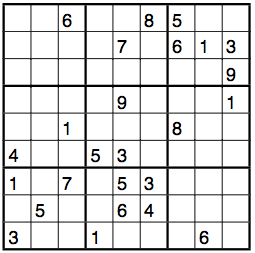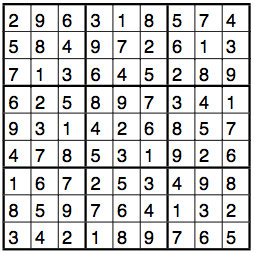Mathematics and Sudokus: Solving Algorithms (I)

In this section, we will explore algorithms that solve Sudoku puzzles. A key aspect of an algorithm is that it terminates. For a Sudoku solving algorithm, that means that the procedure will eventually end and tell us if a given Sudoku has a solution, and if yes, then we want to know at least one solution (there could be many). Note that “looking at the puzzle and trying to figure it out” would not qualify as an algorithm, since the solver might never finish.

The “Simple Solving Algorithm”

Our Sudoku counting methods from an earlier section suggest a very simple Sudoku solving algorithm. Before you read on, think a couple of minutes and try to come up with it yourself.

Here is the first solving algorithm. Since it’s very simple, we will call it the “Simple Solving Algorithm”. We start with a partially filled Sudoku board, and assume for simplicity’s sake that the Sudoku is not yet complete.

1. 1.Enumerate all empty cells in typewriter order (left to right, top to bottom)

2. 2.Our “current cell” is the first cell in the enumeration.

3. 3.Enter a 1 into the current cell. If this violates the Sudoku condition, try entering a 2, then a 3, and so forth, until
a. the entry does not violate the Sudoku condition, or until
b. you have reached 9 and still violate the Sudoku condition.

4. 4.In case a: if the current cell was the last enumerated one, then the puzzle is solved. If not, then go back to step 2 with the “current cell” being the next cell.
In case b: if the current cell is the first cell in the enumeration, then the Sudoku puzzle does not have a solution. If not, then erase the 9 from the corrent cell, call the previous cell in the enumeration the new “current cell”, and continue with step 3.

If you know MATLAB (or any imperative programming language), you might like look at my MATLAB implementation of the algorithm. It’s not optimized for speed, but for readability. The code comes in two parts: anydouble.m, which checks whether there are any numbers that appear twice in any row, column or 3x3 box, and solve_sudoku.m, which is the main file. To solve a Sudoku puzzle, download the two files, enter the Sudoku matrix that you want the algorithm to solve at the top of solve_sudoku.m (an example for the formatting is included in the file), save, and run solve_sudoku.m.

I tested my code using the following puzzle. It’s the first puzzle from www.veryfreesudoku.com/printablesudokupuzzles/Fiendish-Book1.pdf . Its rating is “fiendish”.The Sudoku solver took about 5 minutes to come up with the following solution:Activity 6  (optional, requires some programming knowledge): Implement the simple solving algorithm in a programming language of your choice. Matlab has a lot of useful built-in functions that would help you (for example the “find” function), but this is also a fun exercise if you have a basic knowledge of C, Java, or even some functional programming language.

Activity 7: Explain in a paragraph or two why our algorithm will find a solution to any Sudoku puzzle with a solution. Also explain how we could modify the algorithm to find every solution to a given puzzle.

Two simple-minded approaches

The previous activity suggests that we have found a solving algorithm that is in theory very useful. In practice, it turns out that the algorithm is quite slow: my own implementation in Matlab was about as fast as a human on most puzzles, sometimes a lot slower. Certainly nothing I could impress my dad with. Something else is annoying about this algorithm: it’s virtually impossible to do on paper, there are just too many numbers that need to be entered and erased.

We will now look at some solving methods that work well on paper. Our ultimate goal is to introduce an algorithm that allows a person to solve any Sudoku puzzle on paper, or say (with confidence!) that there is no solution.

When you did your first Sudoku puzzle, you probably noticed that you can solve some Sudoku puzzles by taking each number from 1 to 9 in turn and by observing that a certain field can only contain a certain number. In other words, you check which numbers may go in a certain cell. If there is only one candidate, then you enter that number. It is natural to go through all cells in typewriter order and make this check, and if possible add enter the number in the corresponding field. Some puzzles can be solved in this way. However, there might be a point where you get stuck with this method: once you have considered each cell at least once since last entering a number, you can be sure that this method will not solve the puzzle for you. Let’s call this method the candidate-checking method.

There is another method that immediately comes to mind to the beginner Sudoku solver: look at a particular column (or row, or box). You know that each column, row and box must contain the number 1 at the end. Check in how many places you can enter the number 1 without violating the Sudoku condition. If there is only one such cell, then you can enter the 1 in that cell. Now do the same check for the number 2. Once you are done with all numbers up to 9, repeat the process with the next column. Once you are done with all columns, do the rows, and then the boxes. As with the previous method, there are puzzles which cannot be solved using only this method: once you have considered each column, row and box at least once since being able to enter a number,  you will not make any more progress using this method alone. Since this method involves finding a place for each number, we will call this the place-finding method.

Having run through the place-finding method once, you can go back to the candidate-checking method: if you have filled some more cells since last trying the first method, there is a chance that it will now allow you to fill some more cells. Once you get stuck again, try the place-finding method again. Switching back and forth between the two place-finding method and the candidate-checking method will enable you to solve many of the Sudoku puzzles that you find in newspapers and magazines, and it’s a relatively fast way of solving Sudokus.

Activity 8: Find a Sudoku puzzle in a magazine or online (google “Sudoku free”) that is labelled “hard”. Try using the approach described in the previous paragraph (and no other methods!) to solve the puzzle. Hint: Remember that if you have looked at all columns, rows and 3x3 boxes once since last making any progress with either of the two methods, you can be confident that switching between candidate-checking and place-finding will not solve the puzzle.